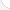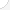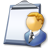ActuariesDescription: Actuaries design insurance plans that will help their company make a profit. They study statistics and social trends to decide how much money an insurance company should charge for an insurance policy. They predict the amount of money an insurance company will have to pay to its customers for claims. Some actuaries are selfemployed and work as consultants.Source: Bureau of Labor Statistics, U.S. Department of Labor, Occupational Outlook Handbook, 2008-09 Edition at http://www.bls.gov/OCO/Complete Job Profile: http://www.bls.gov/oco/ocos041.htmSalary: \$50,001 or more per yearComments:Actuaries need mathematics beyond calculus and all these topics are necessary background to succeed in these college classes. sormanic@member.ams.org christina sormani

There are 71 math topics Actuaries need to know.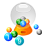Basic Math / Algebra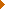FractionsDecimalsRatio and ProportionPercentCustomary MeasurementMetric MeasurementMeasurement ConversionBasic ProbabilityBasic StatisticsStatistical GraphingPowers and RootsOther Number BasesNegative NumbersScientific NotationBasic Problem Solving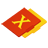First-Year AlgebraUsing FormulasLinear EquationsLinear InequalitiesOperations with PolynomialsFactoring PolynomialsRational ExpressionsCoordinate Graphing 2DLinear SystemsRadicalsQuadratic EquationsAlgebraic Representation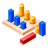GeometryBasic TerminologyAngle MeasurementCongruent TrianglesTriangle InequalitiesParallel LinesQuadrilateralsSimilarityGeometric MeanPythagorean TheoremRight Triangle TrigonometryCirclesConstructionsAreaVolumeTransformationsMake/Use 3D Drawings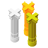Second-Year Algebra / TrigonometryFunctionsVariationImaginary NumbersPolynomial EquationsLogarithmsSequences and SeriesMatricesCoordinate Graphing 3DAdvanced ProbabilityAdvanced StatisticsConic SectionsNon-Linear SystemsTrigonometric/Circular FunctionsGraphs of Trigonometric FunctionsTrigonometric IdentitiesTrigonometric Equations/InversesOblique TrianglesPolar Coordinates/GraphsVectors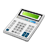Other TopicsCalculus and Higher MathBasic Calculator UseScientific Calculator UseComputer UseComputer ProgrammingGroup Problem SolvingMental MathInductive/Deductive ReasoningMath CommunicationsMathematical Modeling